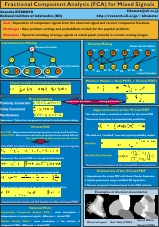# 1. Summary

The problem of reconstructing component signals from the observed mixed signal is a problem found in many scientific disciplines, and hence has the wide range of applications. The contribution of this research, namely Fractional Component Analysis (FCA), is to establish a stochastic model for the case where component fractions are unity convex combination and stochastic. We also demonstrate that our stochastic model leads to an interesting probability distribution function, and this function is useful for the modelling of, for example, remote sensing images.

# 2. Fractional Component Analysis (FCA)The goal of the "Fractional component analysis (FCA)" is to analyze the observed signal generated by the linear mixing process of unknown fractional component signals. Based on simple assumptions on the probability distributions of component signals and fractions, we derive a new type of distribution, which we call "blended distribution," and characterize this model in terms of moments and cumulants. Its higher-order cumulants indicate that it is in fact either super-gaussian or sub-gaussian even if the distribution of component signals are gaussian. Finally we show methods for recovering fractional components from the observed signal. The background of this motivation is in the problem of "mixed pixel (mixel) analysis."

# 3. References (Complete List)

1. Asanobu KITAMOTO, "Fractional Component Analysis (FCA) for Mixed Signals", Proceedings of the 16th International Conference on Pattern Recognition (ICPR'02), Vol. 3, pp. 383-386, IEEE, doi:10.1109/ICPR.2002.1047925, 2002-8 [ Abstract ] [ Paper ]
2. Asanobu KITAMOTO, "FCA: The Fractional Component Analysis", Proceedings of the 4th Workshop on Information-Based Induction Sciences (IBIS 2001), pp. 297-302, 2001-8 [ Abstract ] [ Paper ]